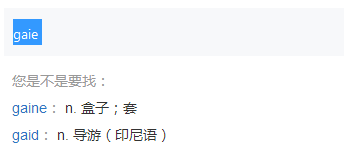# 拼写检查编程题详解-BK树算法

2018/11/12 00:09

BK树：

C++AC代码：

## 问题：1035:拼写检查

1、删除单词A的一个字母后得到单词B；

2、用任意一个字母替换单词A的一个字母后得到单词B；

3、在单词A的任意位置增加一个字母后得到单词B。

i
is
has
have
be
my
more
contest
me
too
if
award
#
me
aware
m
contest
hav
oo
or
i
fi
mre
#

me is correct
aware: award
m: i my me
contest is correct
hav: has have
oo: too
or:
i is correct
fi: i
mre: more me

## 分析：### BK树：

BK树或者称为Burkhard-Keller树，是一种基于树的数据结构，被设计于快速查找近似字符串匹配，比方说拼写纠错，或模糊查找，当搜索”aeek”时能返回”seek”和”peek”。BK树在1973年由Burkhard和Keller第一次提出，论文在这《Some approaches to best match file searching》。这是网上唯一的ACM存档，需要订阅。更细节的内容，可以阅读这篇论文《Fast Approximate String Matching in a Dictionary》。

1. d(x,y) = 0 当且仅当 x=y         （Levenshtein距离为0 <==> 字符串相等）

2. d(x,y) = d(y,x)                        （从x变到y的最少步数就是从y变到x的最少步数）

3. d(x,y) + d(y,z) >= d(x,z)         （从x变到z所需的步数不会超过x先变成y再变成z的步数）

### 查询相似词：

BK树是多路查找树，并且是不规则的（但通常是平衡的）。试验表明，一次查询所遍历的节点不会超过所有节点的5%到8%，两次查询则一般不会17-25%，效率远远超过暴力枚举。适当进行缓存，减小Levenshtein距离常数n可以使算法效率更高。需要注意的是，如果要进行精确查找，也可以非常有效地通过简单地将n设置为0进行。

### 推论：

d(gaie, B) + d(B, A) >= d(gaie, A),  即 d(gaie, B) + d(A,B) >= d

-->  d(A,B) >= d - d(gaie, B) >= d - n

d(A, B) <= d(A,gaie) + d(gaie, B),   即 d(A, B) <= d + d(gaie, B) <= d + n

--> d(A,B) >= d(gaie, B) - d(gaie, A)

--> d(A,B) >= 1 - d >= 0 (gaie与B不等) 由于 A与B不是同一个字符串，所以d(A,B)>=1

## C++AC代码：

#include <iostream>
#include <string>
#include <map>

using namespace std;
struct nodew{             //存储单词信息，因为POJ要求输出相似词时，需按照读入顺序输出，所以得设置单词的顺序
int order;
string word;
};

typedef struct treeNode{                    //结构体，存储树节点，我这里使用map容器，没有使用指针，性质差不多。但map会节省点空间。
struct nodew word;
map<int, struct treeNode*> bkTreeNode;  //map容器存储孩子节点，map的key对应编辑距离，value值对应结点。
}BKTreeNode;
bool isSame = false;                        //标识两个单词是否相同
map<int,string> result;                     //存储距离为1时的结果集

int levenSTDistance(string x, string y);                   //计算字符串x和字符串y的levenshtein距离
int minOfTreeNum(int a, int b, int c);                     //返回a，b，c三个数中最小值
void queryWord(BKTreeNode* tNode, string word);            //查询单词是否在字典，如果不在的话返回距离为1的结果集
void buildTree(BKTreeNode* tNode, struct nodew nodBuild);  //构造BK树
BKTreeNode* newTreeNode();                                 //初始化节点。

int main()
{
string dic;                        //词典单词
string word;                       //待查询单词
//vector<string>::iterator vIter;  //一开始没有考虑顺序，所以换为了map
map<int,string>::iterator mIter;   //map容器的迭代器
string temp;   //中间变量
int cnt = 1;   //为字典中单词编号，排顺序

cin >> dic;
BKTreeNode* root = newTreeNode();
struct nodew nodetemp;     //单词结构体，初始化完成后再存入词典
nodetemp.word = dic;
nodetemp.order = 0;        //为树根单词编号为0
root->word = nodetemp;
while(1)
{
cin >> dic;
if(dic == "#")
{
break;
}
nodetemp.order = cnt++;    //为单词标记读入顺序
nodetemp.word = dic;       //初始化单词
buildTree(root,nodetemp);  //构造BK树
}
while(1)
{
cin >>word;
if(word == "#")
{
break;
}
isSame = false;        //每查完一个单词之后置为false
result.clear();        //将结果集清空
queryWord(root,word);  //在BK树中查找满足要求的单词
if(!isSame)            //如果字典中没有找到，则输出相似单词
{
cout << word << ":";
for(mIter = result.begin(); mIter != result.end(); mIter++) //迭代输出结果集
{
temp = mIter->second;
cout << " " << temp;
}
}else{
cout << word <<" is correct" ;    //在词典中找到的话，直接输出正确。
}
cout << endl;
}
return 0;
}

//初始化节点
BKTreeNode* newTreeNode()
{
BKTreeNode * node = new BKTreeNode;
return node;
}

void buildTree(BKTreeNode* tNode, struct nodew nodBuild) //构造BK树
{
string newNode = nodBuild.word;                   //新进来的结点
string dicNode = tNode->word.word;                //BK树中的节点
int distance = levenSTDistance(newNode, dicNode); //计算编辑距离
map<int,BKTreeNode*>::iterator iter;              //BK树子树迭代器

iter = tNode->bkTreeNode.find(distance);          //查找孩子树种是否存在
if(iter != tNode->bkTreeNode.end())               //存在的话迭代插入孙子结点
{
buildTree(iter->second, nodBuild);
}else{                                            //不存在的话插入孩子结点
BKTreeNode* tempNode = newTreeNode();
tempNode->word = nodBuild;
tNode->bkTreeNode[distance] = tempNode;
}
}

void queryWord(BKTreeNode* tNode, string word)
{
string dicNode = tNode->word.word;
int ord;
int distance = levenSTDistance(dicNode, word);
int i;
map<int, BKTreeNode*>::iterator iter;

if(distance == 0)
{
isSame = true;
}else{
if(distance == 1){
ord = tNode->word.order;
result[ord] = dicNode;
}
i = max(1,distance-1);
for(; i <= distance+1 ; i++)
{
iter = tNode->bkTreeNode.find(i);
if(iter != tNode->bkTreeNode.end())
{
queryWord(iter->second, word);
}

}
}
}

int minOfTreeNum(int a, int b, int c)  //返回a，b，c三个数中最小值
{
int minNum = a;
if(minNum > b )
{
minNum = b;
}
if(minNum > c )
{
minNum = c;
}
return minNum;
}

int levenSTDistance(string x, string y)  //计算字符串x和字符串y的levenshtein距离
{
int lenx = x.length();
int leny = y.length();
int levenST[lenx+1][leny+1];  //申请一个二维数组存放编辑距离
int eq = 0;                   //存放两个字母是否相等
int i,j;

//初始化二维数组，也就是将最简单情形的levenshtein距离写入
for(i=0; i <= lenx; i++)
{
levenST[i] = i;
}
for(j=0; j <= leny; j++)
{
levenST[j] = j;
}

//将串x和串y中的字母两两进行比较，得出相应字串的编辑距离
for(i=1; i <= lenx; i++ )
{
for(j=1; j <= leny; j++)
{
if(x[i-1] == y[j-1])
{
eq = 0;
}else{
eq = 1;
}
levenST[i][j] = minOfTreeNum(levenST[i-1][j] + 1, levenST[i][j-1] + 1, levenST[i-1][j-1] + eq);
}
}
return levenST[lenx][leny];
}


0
0 收藏

### 作者的其它热门文章0 评论
0 收藏
0